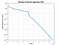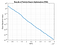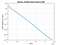# Introduction

Evolutionary Algorithms (EAs) and Metaheuristics are general-purpose tools to deal with optimization problems, mostly having a black-box objective function. These algorithms are considered as a subfield of Computational Intelligence (CI) and Artificial Intelligence (AI), and they have enormous applications in many fields of science and engineering. Evolutionary algorithms are nature-inspired and they are mostly simulating what happens in natural evolution to come up with approximate solutions for optimization problems, just like the way nature has represented us and other living organisms as solutions for the problem of living on Earth.

1. Creating New Solutions: Based on the current population, and using processes like competition, cooperation, selection, breeding, mutation, etc., some new solutions (aka. offsprings) are created. The set of newly created solutions can be combined with current (parent) solutions in various ways. The result, maybe after a selection and competition process, will be the new current population.
2. Checking for Termination: Check if there is an acceptable solution. If an acceptable solution has been found, then execution of the algorithm terminates, otherwise, the algorithm continues from step 2.
• Performing crossover (similar to reproduction)
• Performing mutation (similar to natural mutation)
• Combine population of parents and offsprings
• Perform competition among members of the combined population
• Select members of the new generation
• The new position is evaluated.
• The best memory of the particle (aka. personal best) is updated.
• The best collective memory of the whole swarm (aka. global best) is updated.

# Yarpiz Evolutionary Algorithms Toolbox

Within the last few years, I have shared many codes on Yarpiz, which implement various AI, Machine Learning, and Computational Intelligence methods, algorithms, and applications. Most of the projects are developed in MATLAB and they are also available on MATLAB File Exchange (FEX) to download.

• 8 real-valued variables in the range [-5, 5],
• 2 integer variables from set {3, 4, 5, 6},
• a 3-by-5 matrix of real-valued variables in range [0, 2],
• 5 permutations of length 20,
• a segmentation of 30 elements into 7 disjoint subsets, and
• selection of 6 out of 20 members of a set.
• After the variables are defined, the user can implement the objective function, using real-world variables. This makes the implementation of objective function much easier.
• It implements several metaheuristic algorithms with similar standards, and this makes it easier to apply multiple algorithms to an optimization problem without the need to modify the code. So the problem is defined once, but it can be solved multiple times by different optimizers.

## List of Algorithms

Currently, 15 metaheuristics and evolutionary algorithms are implemented in the YPEA toolbox. The list of implemented algorithms and a short description of each algorithm follows. The list is orderer alphabetically, according to the full name of the algorithms.

1. Ant Colony Optimization for Continuous Domains (ACOR)
Ant Colony Optimization (ACO), proposed by Marco Dorigo in 1992, is mainly used for discrete and combinatorial optimization problems. Socha and Dorigo proposed the continuous version in 2008, which uses a probabilistic model to find a model for better solutions.
2. Bees Algorithm (BA)
This is another algorithm inspired by the intelligent foraging behavior of honey bees and it is proposed by Professor Duc Truong Pham et al. in 2005.
3. Biogeography-based Optimization (BBO)
Originally developed by Dan Simon in 2008, this algorithm is based on the biogeography and distribution of species in various habitats. BBO simulates the process of immigration and emigration between territories.
4. Covariance Matrix Adaptation Evolution Strategy (CMA-ES)
Evolution Strategy (ES) is a well-known evolutionary algorithm and its development dates back to the early 1960s. Up to now, many variations of ES has been proposed. CMA-ES is a probabilistic and enhanced version of ES and it is proposed by Nikolaus Hansen in the 2000s.
5. Cultural Algorithm (CA)
Proposed by ‪Robert G. Reynolds in 1994, cultural algorithms use the concept of culture, knowledge, and belief space in addition to individuals to perform evolution. In this algorithm, a belief space is used to model the common experience of the whole population. Cultural Algorithm can be considered as a sample of Social Darwinism.
6. Differential Evolution (DE)
This algorithm uses the differences of individuals in the population to create new candidate solutions. There are various schemes to create new solutions, hence, DE has various versions and modifications. Differential Evolution is proposed by Rainer Storn and Kenneth Price in 1995.
7. Firefly Algorithm (FA)
This algorithm is proposed and developed by Xin-She Yang in 2009 and it is inspired by the fireflies and their flashing behavior.
8. Genetic Algorithm (GA)
Simulation of natural evolution was started in the early 1950s. At that time, 3 separate groups were working on the idea of creating an intelligent general-purpose tool to solve every kind of problem, especially optimization problems. The first approach resulted in the development of the Evolution Strategy (ES), the second one caused the introduction of the Evolutionary Programming (EP), and the third approach ended up with the introduction of GA. John Holland studied genetic algorithms and through his work, GAs became popular in the early 1970s.
9. Harmony Search (HS)
This algorithm is inspired by the process of music composition and the approach musicians use to find better harmonies in music. Harmony Search is proposed by Zong Woo Geem et al. in 2001.
10. Imperialist Competitive Algorithm (ICA)
This algorithm is a socio-political metaheuristic, inspired by the historical colonization process and competition among imperialists, to capture more and more colonies. ICA is developed and proposed by Esmaeil Atashpaz Gargari and Caro Lucas, in 2007.
11. Invasive Weed Optimization (IWO)
This algorithm is proposed by Alireza Mehrabian and Caro Lucas, in 2006. IWO imitates the spreading strategy of weeds, which can be described using r/K Selection Theory.
12. Particle Swarm Optimization (PSO)
PSO is inspired by the social behavior of animal groups, like fish schools and flocks of birds. James Kennedy and Russell C. Eberhart originally intended to simulate the social behavior of animals. But they ended up proposing a new optimizer algorithm in 1995, which is currently known as PSO.
13. Simulated Annealing (SA)
While this algorithm is developed and proposed by several independent groups of researchers, for the first time, Scott Kirkpatrick et al. used SA to solve the Travelling Salesman Problem (TSP), in 1983. Simulated Annealing is inspired by the annealing process in metallurgy, which is a heat treatment operation to make the materials, especially metals, more flexible and strong.
14. Teaching-Learning-based Optimization (TLBO)
TLBO simulates the process of teaching, learning, and passing knowledge in a classroom. The main source of learning is the teacher. However, students also can learn by interacting with each other. This algorithm is proposed by Ravipudi Venkata Rao et al. in 2011.

## List of Supported Variable Types

One of the difficulties in modeling and defining optimization problems is dealing with different kinds of decision variables. In YPEA, there several types of decision variables are supported and make the problem definition much easier.

• Integer Variables, which are used to model integer-valued (discrete) variables, in any size (scalar, vector, matrix, etc.), with any combination of lower and upper bounds.
Example: Generalized Knapsack Problem.
• Binary Variables, which are special cases of integer variables, with lower bound set to 0, and upper bound set to 1.
Example: Standard (Binary) Knapsack Problem.
• Permutation Variables, which are used to model permutations of some items, and can include more than one permutation per variable (as rows of a matrix).
Example: Traveling Salesman Problem (TSP), n-Queens Problem, and Quadratic Assignment Problem (QAP).
• Selection Variables, which are used to model fixed-length selections of some items, and can include more than one selection per variable (as rows of matrix).
Example: Feature Selection.
• Partition/Allocation Variables, which are used to partition a list of items into disjoint subsets (also known as allocation and assignment), and can include more than one partitioning per variable (as rows of matrices).
Example: Vehicle Routing Problem (VRP) and Hub Location Allocation.
• Fixed Sum Variables, which are used to model real-valued variables that are needed to have some fixed target sum value (in the whole matrix, in rows, or in columns).
Example: Transportation Problem and Supply Chain Management.

The source code of this YPEA toolbox is shared on GitHub, via this URL [+]. If you want to install the toolbox directly, you can download the installable MATLAB Toolbox File (*.mltbx), from this link [+].

`doc ypea`

# How YPEA Works?

The main steps to set up your problem and solve it using YPEA are listed below:

• Set the type of optimization problem (either minimization or maximization)
• Define search space and decision variables
• Define the objective function
• Note: In the case of constrained optimization problems, constraints are defined and using methods like penalty functions, they are incorporated into the final objective function.
• Set the parameters of algorithms
• Call `solve` method of the algorithm, passing the problem object into it
• Get the optimization results and visualize (if it is needed)

# Solving a Classic Problem

As a simple problem, we are going to optimize the well-know ‘Sphere’ function, which is defined as follows:

## Problem Definition

Now we will define the problem structure, specifying the type of problem, the search space, and the objective function. So we have this code to define the problem structure:

## Using Genetic Algorithm

The class `ypea_ga` in the toolbox defines the Real-coded Genetic Algorithm. The code below shows how we can use the GA to solve our optimization problem. First, we need to create an instance of ypea_ga and then, we will use it to get the problem solved.

`Genetic Algorithm started ...Initializing population.Iteration 1: Best this. Value = 329.689, NFE = 200Iteration 2: Best this. Value = 313.7412, NFE = 300Iteration 3: Best this. Value = 240.6908, NFE = 400Iteration 4: Best this. Value = 232.9948, NFE = 500Iteration 5: Best this. Value = 152.2398, NFE = 600...Iteration 995: Best this. Value = 1.5244e-09, NFE = 99600Iteration 996: Best this. Value = 1.5244e-09, NFE = 99700Iteration 997: Best this. Value = 1.5244e-09, NFE = 99800Iteration 998: Best this. Value = 1.5243e-09, NFE = 99900Iteration 999: Best this. Value = 1.5243e-09, NFE = 100000Iteration 1000: Best this. Value = 1.5243e-09, NFE = 100100End of optimization.`
`>> best_sol.solutionans =  struct with fields:    x: [1×20 double]`
`>> best_sol.solution.xans =     0  0  0  0  0  0  0  0  0  0  0  0  0  0  0  0  0  0  0  0`
`>> best_sol.obj_valueans =     0`
`>> ga.run_timeans =    8.9737`
`>> ga.nfeans =      100100`Convergence plot of GA, illustrating the best objective value vs. the number of function evaluations

## Using Particle Swarm Optimization

Once a problem is defined in the YPEA format, it is possible to solve it using any other algorithm implemented and available in the toolbox. For example, instead of using GA, we can use PSO to solve the problem of finding the global minimum of the Sphere function as follows. Also, a sample of the output plot is shown after the code.Convergence plot of PSO, illustrating the best objective value vs. the NFE

## Using Differential Evolution

Similarly, we can use Differential Evolution to solve the problem we have defined above. According to the conventions found in the literature of DE, we are going to use the DE/best/2/exp version, which means:

• two vector differences are used (i.e. four random solutions contributing to the creation of mutated vector), and
• exponential crossover is utilized.
• DE/rand-to-best/1/exp
• DE/target-to-best/3/bin
• DE/best/1/bin
• DE/rand/5/expConvergence plot of DE, illustrating the best objective value vs. the NFE

# Conclusion

YEPA toolbox provides a unified approach to define optimization problems and solve them using various metaheuristics and evolutionary algorithms. This makes the process of research or developing new applications in the field of optimization much easier. Also, once a problem is defined it can be solved with several algorithms without the need to perform any modifications.

# Citing YPEA

YPEA Toolbox for MATLAB is open-source and free to use. If you are going to use the toolbox in your research projects and you want to cite that, please use the following format.